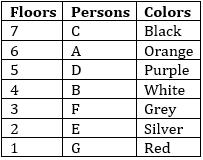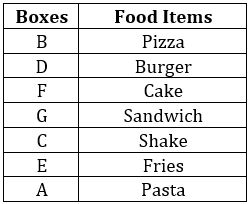Latest Banking jobs   »

# Reasoning Ability Quiz For LIC AAO 2023- 25th January

Directions (1-5): Study the information given below and answer the given questions.
There are seven persons A, B, C, D, E, F and G live on seven floors building, but not necessarily in the same order. Only one person lives on each floor. All of them like different colors i.e., red, orange, purple, black, white, silver and grey, but not necessarily in the same order.
D lives on an odd number floor above the floor number 3. There are two floors between the floor on which D lives and the floor of the one who like silver color. E lives on an even number floor and lives immediately below F. The person who lives on topmost floor likes black color and he is not F. D does not like Orange and white color. There are two floors between the floor of the one who like Orange and the one who like grey color and neither of them lives on bottom most floor. G likes red color and lives below B who live on an even number floor below the floor number 6. F lives on an odd number floor below 5th floor. There is one floor gap between the floors on which D and C live. Neither C nor D likes orange and grey color. E does not like white and purple. A does not like grey color.

Q1. Which colour is liked by the person who live on the 7th floor?
(a) Red
(b) White
(c) Orange
(d) Silver
(e) Black

Q2. How many persons live between the one who likes orange color and the one who lives on 4th floor?
(a) None
(b) One
(c) Two
(d) Three
(e) More than three

Q3. Who lives on the 2nd floor?
(a) The one who has white color
(b) B
(c) A
(d) The one who like silver color
(e) C

Q4. Four of the following five are alike in a certain way and hence they form a group. Which one of the following does not belong to that group?
(a) Red
(b) Black
(c) Purple
(d) Silver
(e) Grey

Q5. Which of the following color does F like?
(a) White
(b) Red
(c) Purple
(d) Grey
(e) Black

Directions: (6-10): In these questions, relationship between different elements is shown in the statements. These statements are followed by three/four conclusions. Read the statements and decide which of the following conclusions follow from the given statements.

Q6. Statements: P<A≤N, P>T, M≤A<S
Conclusions: I. S>P
II. T<A
III. N>T
IV. S>N
(a) Only I and II follow
(b) Only III and IV follow
(c) Only I, II and III follow
(d) Only I and IV follow
(e) None of these

Q7. Statements: P≥R<S≥M>E≥B
Conclusions: I. E<S
II. B≤S
III. M≥S
IV. R=M
(a) Only I follows
(b) Only II and IV follow
(c) Only III and IV follow
(d) Only II follows
(e) None of these

Q8. Statements: G≥M>P, Q≤R>P
Conclusions: I. G>R
II. Q>M
III. P< G
IV. M<R
(a) Only I and III follow
(b) Only II follows
(c) Only III follows
(d) Only II and IV follow
(e) None of these

Q9. Statements: N≥Q>R=B≥S, R>F≥D
Conclusions: I. B>D
II. F≤S
III. N>D
IV. Q>F
(a) Only I, II and III follow
(b) Only I, III and IV follow
(c) Only II, III and IV follow
(d) Only I, II and IV follow
(e) None of these

Q10. Statements: B≥D≥P=Q≥S=K>T≥V
Conclusions: I. B=K
II. D>K
III. V<Q
IV. T<P
(a) Only I and II follow
(b) Only II and III follow
(c) Only III and IV follow
(d) Only I and III follow
(e) None of these

Direction (11-15): Read the following information carefully and answer the given questions:
Seven boxes contain different food items- Cake, Fries, Pizza, Shake, Burger, Pasta and Sandwich are kept above the other, but not necessary in the same order.
More than three boxes are placed in between box of Pizza and Fries. There are two boxes are placed in between Box B and G which contain Sandwich. Box which contains Fries is placed below box of Pizza. Box E is immediately above the box which have Pasta. More than three boxes are placed in between box which contain Pasta and box of Pizza. Box F is immediately below the box of Burger. Shake is contained in box C. Pizza is not packed in box D. The box which contains Fries is immediately above Box A.

Q11. How many boxes are placed in between Box D and the box which contain fries?
(a) Two
(b) One
(c) More than three
(d) Three
(e) None

Q12. Which of the following box contain pizza?
(a) C
(b) F
(c) B
(d) E
(e) A

Q13. Which of the following box is placed just above the box which contain shake?
(a) G
(b) E
(c) A
(d) D
(e) F

Q14. Which of the following food item is packed in box A?
(a) Fries
(b) Pasta
(c) Burger
(d) Pizza
(e) None of these

Q15. How many boxes are placed below the Box C?
(a) None
(b) Two
(c) Three
(d) One
(e) Four

Solutions

Direction (1-5):
Sol.S1. Ans. (e)
S2. Ans. (b)
S3. Ans. (d)
S4. Ans. (d)
S5. Ans. (d)

S6. Ans.(c)
Sol. I.S>A>P(True)
II.S>A>P>T(True)
III.N≥A>P>T(True)
IV.S>A≤N(False)

S7. Ans.(a)
Sol. I.E<M≤S(True)
II.B≤E<M≤S(False)
III.M>S(False)
IV.R<S≥M(False)

S8. Ans.(c)
Sol. I.G≥M>P<R(False)
II.Q≤R>P<M(False)
III.P<M≤G(True)
IV.M>P<R(False)

S9. Ans.(b)
Sol. I. B=R>F≥D(True)
II. F<R=B≥S(False)
III.N≥Q>R>F≥D(True)
IV.Q>R>F(True)

S10. Ans.(c)
Sol. I.B=K(False)
II.D>K(False)
III.V<Q(True)
IV. T<P(True)

Solutions (11-15):
Sol.S11. Ans. (d)
S12. Ans. (c)
S13. Ans. (a)
S14. Ans. (b)
S15. Ans. (b)## FAQs

### When will the LIC AAO Prelims exam be held?

The LIC AAO Prelims exam will be held on 17th & 20th of February 2023 (tentatively).

#### Congratulations!